# Markov chain, recurrent

(diff) ← Older revision | Latest revision (diff) | Newer revision → (diff)
A Markov chain in which a random trajectory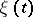, starting at any state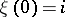, returns to that state with probability 1. In terms of the transition probabilities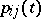, recurrence of a discrete-time Markov chain is equivalent to the divergence for anyof the series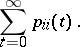In a recurrent Markov chain a trajectory,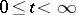,, returns infinitely often to the statewith probability 1. In a recurrent Markov chain there are no inessential states and the essential states decompose into recurrent classes. An example of a recurrent Markov chain is the symmetric random walk on the integer lattice on the line or plane. In the symmetric walk on the line a particle moves from positionto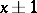with probabilities; in the symmetric walk on the plane a particle moves fromto one of the four points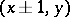,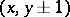with probabilities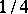. In these examples a particle, starting the walk at an arbitrary point, returns to that point with probability 1. The symmetric walk on the integer lattice in the three-dimensional space, when the probability of transition from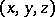to a neighbouring point,,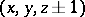is equal to, is not recurrent. In this case the probability of return of the particle to its initial point is approximately 0.35.